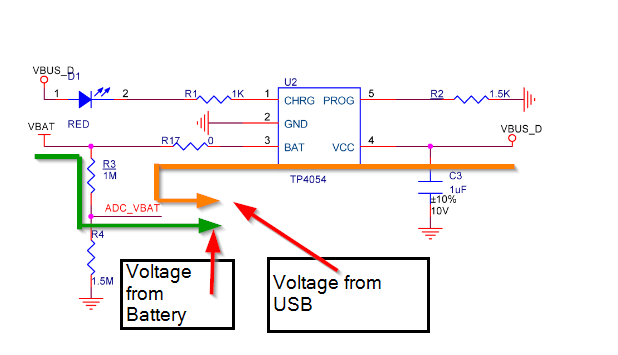# Custom Li-ion battery voltage calculation in RAK4630

In the schematic of WisBlock Base (RAK5005-O) I found it uses 1M ohm and 1.5M ohm voltage divider network by which if we connected a Li-ion battery at full charge (around 4.15V) it will output maximum of 1.6V at ADC_VBAT. Since 4.15 * 0.4 = 1.6V.
I am using 3V internal reference and 12-bit resolution but getting around 2.3V (raw ADC value of around 3235) by the formula adc_raw * (3.0 / 4096). But it should show around 1.6V why is that so? Do I am doing the wrong calculation?

Welcome to RAK forum GaneshCan you share the code you use so we can check?

Sure Carl. Here is the one.

``````void setup() {
Serial.begin(115200);

analogReference(AR_INTERNAL_3_0);
}

void loop() {
float batV = raw_adc * (3.0 / 4096);
Serial.println(batV);

delay(5000);
}``````

Without looking at the schematic, if the 1M is connected to the battery and the 1M5 is on ground, the max would be 2.49V.

You need a multiplier of 0.6.

Thanks got it. And I saw when the battery is removed and it’s connected through USB then it will show the reading. It should be zero right?

Not sure I understand that.

Depending on the schematic - I’ll look at it in the next couple of days

There are three scenarios:

• no battery, USB connected
• meaningless value, you read the Vout of the TP4054 in none-charging mode
• battery, USB connected
• meaningless value, you read the charging voltage of the TP4054
• battery, USB not connectedBut it will never be zero

1 Like

Hi Ganesh,

Nothing is wrong in your code but the compensation on the voltage divider is missing. The `float batV` result that you will have is not the actual battery voltage yet. It is the voltage that you will see on the voltage divider but not the battery voltage.

`float batV = raw_adc * (3.0 / 4096) * 1.73;`

And now you will get the actual battery voltage.

However, if you are using the module and connected to USB. The voltage will be affected by the charger output. If you want to measure the battery voltage only, you need to display it to the LCD or transmit it via BT or LoRa so it will not be affected by the charger IC.

Thanks for the clear explanation. @beegee

@carlrowan Hi Carl how the scaling factor 1.73 is calculated. Can you put some light?

Hi Ganesh,

It is a voltage divider. It is easier for me to do it in paperWhat you get is only the ADC_voltage. The scaling factor is actually 1.666666667. I just rounded it off to 1.7.Yes I know its easy but you mentioned 1.73V right so I am wondering where the 0.03V come from? Now its clear thanks for the inputs.

Oh. My bad. I though you are asking about the calculationI actually get that (the 1.73) on the default Read_Battery_Level example of WisBlock.

Let me double-check. It can be related to some ADC offset.

Okay Sure @carlrowan

The 1.73 was actually not 100% calculated. But I made real measurements, compared with the internal readings and then adjusted the scaling factor.
1M ±1%
1.5M ± 1%
ADC of nRF52 has tolerances as well.

1.73 was taken after measuring with several boards and MCU`s.

1 Like

Ideally @newuser_rak4630 , if you want accurate readings from the ADC, you need to calibrate it even via a simple linear equation using a calibrated multimeter as reference. But if you’ll just measure the battery voltage, either 1.73, 1.67 or 1.7 should be workableOkay I am planning to go with 1.67V thanks for your inputs on this.